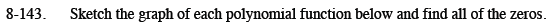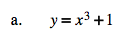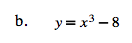Home > CCA2 > Chapter 8 > Lesson 8.3.2 > Problem8-143

8-143.
1. Sketch the graph of each polynomial function below and find all of the zeros. Homework Help ✎

1. y = x3 + 1

2. y = x3 − 8The parent graph is y = x3. How is it shifted?

This function has one x-intercept, which is a zero of the function. Divide the polynomial by the associated factor to obtain a quadratic factor. Then use the Quadratic Formula to obtain the other two (complex) zeros.$x=2, -1\pm i\sqrt{3}$